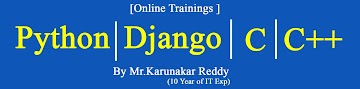-->### Microsoft-Excel 2007 Study Material Day-twenty Five

VLOOKUP Function :
Use VLOOKUP, one of the lookup and reference functions, when you need to find things in a table or a range by row.

Syntax :
=VLOOKUP(lookup_value,table_array,col_index_num,[range_lookup])

Description:
=VLOOKUP(Value you want to look up, range where you want to lookup the value, the column number in the range containing the return value, Exact Match or Approximate Match – indicated as 0/FALSE or 1/TRUE).